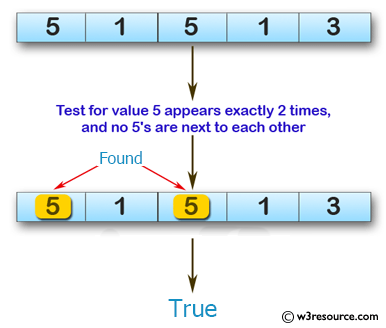﻿ Swift Array Programming Exercise: Test if the value 5 appears in a given array of integers exactly 2 times, and no 5's are next to each other - w3resource# Swift Array Programming Exercises: Test if the value 5 appears in a given array of integers exactly 2 times, and no 5's are next to each other

## Swift Array Programming: Exercise-35 with Solution

Write a Swift program to test if the value 5 appears in a given array of integers exactly 2 times, and no 5's are next to each other.

Pictorial Presentation:Sample Solution:

Swift Code:

``````func test_5(array_nums: [Int]) -> Bool {
var ctr = 0

for num in array_nums {
if num == 5 {
ctr += 1
}
}

for x in 0..<array_nums.count-1 {
if array_nums[x] == 5 && array_nums[x+1] == 5
{
return false
}
}

return ctr == 2
}

print(test_5(array_nums: [5, 1, 5, 1, 3]))
print(test_5(array_nums: [5, 1, 5, 5]))
print(test_5(array_nums: [5, 4, 5, 5, 4]))
```
```

Sample Output:

```true
false
false
```

Swift Programming Code Editor:

Improve this sample solution and post your code through Disqus

What is the difficulty level of this exercise?

﻿